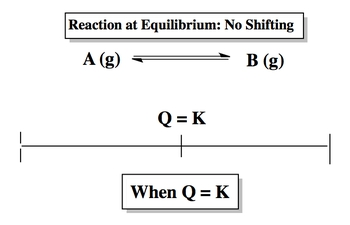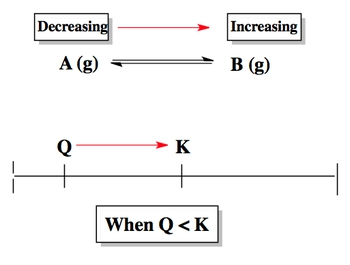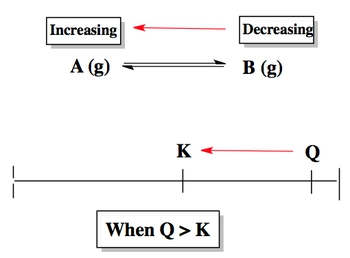## General Chemistry

Learn the toughest concepts covered in Chemistry with step-by-step video tutorials and practice problems by world-class tutors

16. Chemical Equilibrium

# The Reaction Quotient

The reaction quotient, Q, is used to determine if our chemical reaction is at equilibrium.

Equilibrium Determination

Depending on if Q is greater than or less than K our reaction will shift to attain equilibrium by reaching the equilibrium constant K. The direction our reaction shifts determines whether our reactants or products are increasing or decreasing.

1
example

## Q vs. K5m
Play a video:
Hey, guys. So here we're dealing with a new concept. The reaction quotient, which is represented by Q. Now we're gonna say we've learned thus far that any given chemical reaction of equilibrium has an equilibrium constant k associated with it. Now we're looking at Kew, which is our reaction quotient. It's used to determine if our chemical reaction is at equilibrium. Now we're going to say, if the reaction question Q is equal to the great Khan, the equilibrium, constant K and our reaction is at equilibrium. But what if our Q and care not equal to each other? What happens then? Well, let's say that our Q is greater than K here. We're gonna make up Ah, fictitious equation. So a plus B gives me C plus deep, and once they hear that K is equal to 10 but Q was equal to 150 to deal with this. All we do is we do a number line. Que is where we wanna be Equilibrium que, however, is larger. Our equation will shift to try to get back to equilibrium. So which way does Q have toe walk to get to K? Well, he was gonna have to shift this way to get to catch and the same direction. Accused shifts To get two K is the same direction that our equation will shift to get back to equilibrium. So our equation will shift in the same direction. Whichever where we shift that side will be increasing. So this side here is increasing. And if this side is increasing, what's happening to the opposite side? The opposite side would have to be decreasing. Now let's look at the opposite. What if Q is smaller than K? Come up with the same exact idea? So I will say again, a plus B gives me C plus deep now K is still 10. But now we're gonna say Que was equal 2. do a number line. Okay, again is in the middle where we wanna be. The queue is smaller. Which way does Q have to shift to get to K? Que has to shift this way now. Whichever way it shifts on the number line is the same direction shifts in our equation. So we'll shift that way and as a result, wherever we're shifting increases and then this side here is decreasing and That's the approach you need to take. If Cuba is equal to K, they're both on the same exact spot on the number line, so there was no shifting that occurs. Knowing this, let's take a look at this question. It says here for the reaction to H two s gives us hte to plus s to the equilibrium. Constants. Okay, is equal to 1.60 times 10 to the negative, too, at 400 Kelvin temperatures just given. But it's not necessary here. If the reaction question, which is Q has given us 4.18 times tension negative four. Which Wilfong statements is are not true. So do a number line case in the middle what we wanna be. It's to the negative, too, whereas Cuba's to the negative four. So cue was definitely smaller. Say to yourself, which way does Q have to shift to get to equilibrium? Q. S to shift this way to get to equilibrium, Therefore, our equation shift that same way. Remember, wherever we shift will be increasing and therefore the other side will be decreasing. So here, if we take a look, the pressure of s to increase all right. So these are all gasses. When the amount of a gas increases, its partial pressure or pressure will increase. If it's amount decreases, then the gas is pressure will decrease. So we're shifting to the right, which is the site of S two. So s two will be increasing. This is true. The pressure of H two will decrease. No, we're shifting to the side of H two, so it's going to increase, not decrease. Or remember, we're looking for what is not true. The equilibrium constant will not change. That is true. Remember the only thing that can change our equilibrium constant is temperature temperatures not being affected here. So the equilibrium, constant K will stay the same. And here the pressure off H two s will decrease. Yes, because we're shifting away from the react inside, so the react inside would decrease. So based on the options given Onley option, B would be the correct choice. So remember, que was just used as a way of determining. Are we at equilibrium? We're only equilibrium When Q equals K. If they're different, que will have to shift either to the four direction, the reverse direction in order to get back to equilibrium which is kept

If the reaction quotient Q is equal to the equilibrium constant K then our reaction is at equilibrium.When the reaction quotient, Q, is smaller than the equilibrium constant, K, then the reaction shifts in the forward direction to reach equilibrium.When the reaction quotient, Q, is larger than the equilibrium constant, K, then the reaction shifts in the reverse direction to reach equilibrium.2
example

## Determining the Reaction Quotient3m
Play a video:
Hey, guys. So here, if we take a look at this example, it says for the following reaction are equilibrium. Constant is equal to 25. Now. They tell us at a particular time the following concentrations are measured for the given compounds. So each one of these compounds is giving a new concentration, and they're asking us which of the following statements is true. Realize that here they're talking about the amounts of each one of these compounds either increasing or decreasing. And the only way we could know this for sure if it's increasing or decreasing, is to know which way is my reaction shifting. It's shifting towards the product side or the react inside. So what this question is really asking us to do? It's to do Q versus K. Remember. Q. Is your reaction quotient it's used to determine? Are we at equilibrium when Cuba is equal to K were at equilibrium and there will be no shifting, So just like equilibrium, constant Q. Is equal toe products overreact? It's and just like a it ignores solids and liquids. Everything here is a gas, so we're going to keep them around. So we have NH three remember, the coefficient becomes the power. There's a two here, so this is squared. Divided by H two. There's a three year. So this is cube Times end to So What we do now is we're gonna input each of the values for each one of these compounds and federal Q. It's so NH three here is 1.50 times 10 to the negative, too, which is going to be squared, divided by H two, which is 2.25 times 10 to the negative. Six cube times 3.30 times 10 to the negative one. When we punch all that in, it's gonna give us as an answer 5.9 nine times 10 to the 13. Now that we have Q, we can compare it to K to determine which way will our equation shift. So remember K is 25. Que was to the 10 to the 13th Powers. What's much larger? Remember, case what we wanna be? We wanna be an equilibrium. So which way will queue shift? To get to K, you will have to shift to the left so it's gonna go in the reverse direction, which means in our equation. It also goes on the left or reverse direction. Remember, whichever way you're heading will be increasing. And if this side is increasing, the other side has to be decreasing. So now let's see. We're looking for what's true. H two will increase. Yes, that's true because we're heading towards its side here. The equilibrium constant will increase. The only variable that we can use to increase or decrease K is heat. He does not being added or removed, so okay will not change. The concentration of NH three will increase. Now it's decreasing. The concentration of end to will decrease. No, it's increasing. No change will occur only if we're at equilibrium. If Q was equal to K, which we saw that they're not equal, so it does not work. Now that we've seen this, we'll move on to the second example question. You could take a look ahead and see if you can figure it out. If not, don't worry. Just come back and take a look at how I attempt to do this question.
3
example

## Determining Equilibrium Concentrations2m
Play a video: